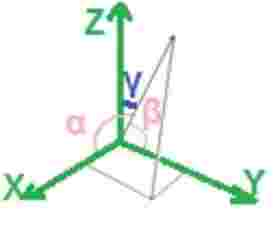# Three Dimensional Vector Angle Calculation

Calculate the angle of three dimensional vectors (3D Vectors) with entered vector coordinates.

 Vector-1 x1 y1 z1 Vector-2 x2 y2 z2

Angle: Degrees

The 3D vectors are using the x-y-z axes.

α is the angle between u and the x-axis.

β is the angle between u and the y-axis.

γ is the angle between u and the z-axis.